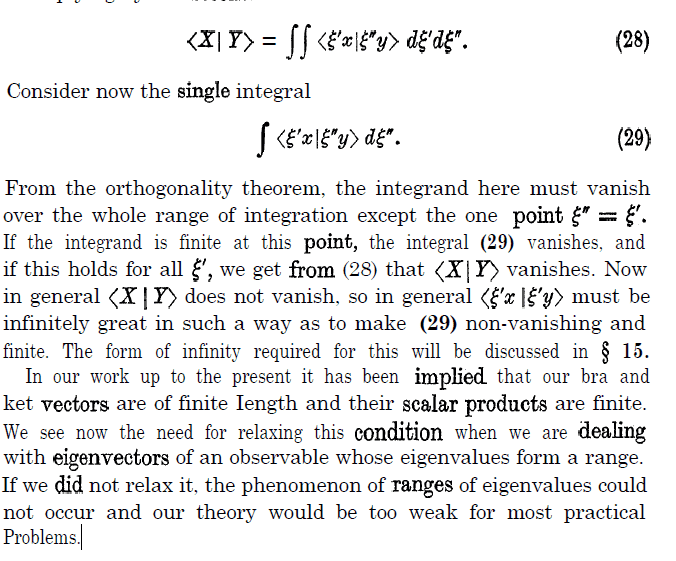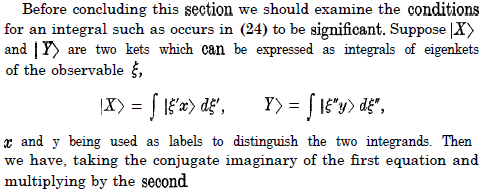# I can't understand this excerpt from a QM book

I cannot understand this excerpt from a book on quantum mechanics with reference to observables.I understand that the orthogonality theorem causes all values except the "equal" values lead to zero.However what do they mean when they say "if the integrand is finite the integral (29) vanishes, and if this holds for all ... <X|Y> vanishes..."
They then claim that in "general <X|Y> does not vanish...

Essentially i cannot garner where such assumptions are formed.Primarily why finite integrals causes the inner product to vanish as well as how it can just be assumed it normally does not vanish.

#### Attachments

PeterDonis
Mentor
a book on quantum mechanics

Which book?

dextercioby
Homework Helper
Which book?

PAM Dirac - The Principles of Quantum Mechanics, 4th edition, page 39.

•bhobba
Klystron
Gold Member
My understanding from https://en.wikipedia.org/wiki/Schur_orthogonality_relations
and the excerpt referring to Section 15 of the (unnamed) textbook [ Dirac?] is that the author made that assumption in order to discuss (teach) infinity forms.

In other words this extract establishes the author's groundwork using finite values before progressing to the more general infinite cases where the bra-ket terms do not vanish.

atyy
I understand that the orthogonality theorem causes all values except the "equal" values lead to zero.However what do they mean when they say "if the integrand is finite the integral (29) vanishes, and if this holds for all ... <X|Y> vanishes..."

If the integrand is zero everywhere except at one point, the integral is zero. See @micromass's replies in this thread:

In the discrete case, for orthogonal basis vectors, the summand is zero except at one point. In the continuous case, the generalization is that for orthogonal basis vectros, the integrand is zero except at one point, where (very loosely speaking) it has to infinite, since an integrand that is finite at one point is still zero when integrated over. This function that is (very loosely speaking) zero everywhere and infinite at one point is called the Dirac delta function. More properly, the Dirac delta function is not a function, and should be called the Dirac delta measure, something that only makes sense when it is ultimately evaluated under an integral
https://en.wikipedia.org/wiki/Dirac_delta_function.
http://homepage.univie.ac.at/reinhold.bertlmann/pdfs/T2_Skript_Chapter_3.pdf (see Eq 3.32 and 3.33)

They then claim that in "general <X|Y> does not vanish...

Here he is referring to the case where ##|X\rangle## and ##|Y\rangle## are not necessarily orthogonal.

Last edited:
•Somali_Physicist and bhobba
bhobba
Mentor
It is Dirac for sure - I recognize it immediately - I have read it so many times

I learnt serious QM from Dirac and Von-Neumann. You hit upon the dreaded Dirac Delta Function issue, Math at the time was not able to handle it, and Von-Neumann being a mathematician was scathing of it. It is thought that he and Hilbert tried to solve it and failed. It took the combined efforts of some later mathematicians, especially Grothendieck, Schwartz and Gelfland to do that.

After my expereince, where I took a sojourn of a few years to look into the solution - its called Rigged Hilbert Spaces - I do NOT recommend starting QM with Dirac or Von-Neumann.

I suggest a more gentle approach - in the following order:
https://www.amazon.com/dp/B06XC7F6QG/?tag=pfamazon01-20
https://www.amazon.com/dp/9332542899/?tag=pfamazon01-20
https://www.amazon.com/dp/9814578584/?tag=pfamazon01-20

If you are more mathematically oriented then look at the following that will explain precisely the Dirac Delta Function and what is going on.:
https://www.amazon.com/dp/0821846302/?tag=pfamazon01-20

But best to do it after Ballentine - QM - A Modern Approach (the third book I suggested) so you fully understand the physios.

Thanks
Bill

Last edited:
•vanhees71 and Somali_Physicist
If the integrand is zero everywhere except at one point, the integral is zero. See @micromass's replies in this thread:

In the discrete case, for orthogonal basis vectors, the summand is zero except at one point. In the continuous case, the generalization is that for orthogonal basis vectros, the integrand is zero except at one point, where (very loosely speaking) it has to infinite, since an integrand that is finite at one point is still zero when integrated over. This function that is (very loosely speaking) zero everywhere and infinite at one point is called the Dirac delta function. More properly, the Dirac delta function is not a function, and should be called the Dirac delta measure, something that only makes sense when it is ultimately evaluated under an integral
https://en.wikipedia.org/wiki/Dirac_delta_function.
http://homepage.univie.ac.at/reinhold.bertlmann/pdfs/T2_Skript_Chapter_3.pdf (see Eq 3.32 and 3.33)

Here he is referring to the case where ##|X\rangle## and ##|Y\rangle## are not necessarily orthogonal.
since an integrand that is finite at one point is still zero when integrated over.
I often see integration as simply as the infinitesimally small steps throughout a curve, hence why wouldnt a bump in the curve simply be added onto the zero of the system?
Here he is referring to the case where ##|X\rangle## and ##|Y\rangle## are not necessarily orthogonal.
By definition ##|X\rangle## and ##|Y\rangle## are orthogonal in all non equal cases.Since both these states correspond to the same observables, why then would he say "generally"

It is Dirac for sure - I recognize it immediately - I have read it so many times

I learnt serious QM from Dirac and Von-Neumann. You hit upon the dreaded Dirac Delta Function issue, Math at the time was not able to handle it, and Von-Neumann being a mathematician was scathing of it. It is thought that he and Hilbert tried to solve it and failed. It took the combined efforts of some later mathematicians, especially Grothendieck, Schwartz and Gelfland to do that.

After my expereince, where I took a sojourn of a few years to look into the solution - its called Rigged Hilbert Spaces - I do NOT recommend starting QM with Dirac or Von-Neumann.

I suggest a more gentle approach - in the following order:
https://www.amazon.com/dp/B06XC7F6QG/?tag=pfamazon01-20
https://www.amazon.com/dp/9332542899/?tag=pfamazon01-20
https://www.amazon.com/dp/9814578584/?tag=pfamazon01-20

If you are more mathematically oriented then look at the following that will explain precisely the Dirac Delta Function and what is going on.:
https://www.amazon.com/dp/0821846302/?tag=pfamazon01-20

But best to do it after Ballentine - QM - A Modern Approach (the third book I suggested) so you fully understand the physios.

Thanks
Bill
I like this book due to the explanation of how to use Bras and Kets.However i do not know if my maths is lacking or dirac is hesistant in going into depth through some parts.For example when arriving in the observable section there seems to be a hesitance to further explain some portions.

atyy
since an integrand that is finite at one point is still zero when integrated over.
I often see integration as simply as the infinitesimally small steps throughout a curve, hence why wouldnt a bump in the curve simply be added onto the zero of the system?

Roughly, the integral is the area under the curve, and the area under a single isolated point is zero. To see this formally, see the posts by micromass in the thread I linked to above.

Here he is referring to the case where ##|X\rangle## and ##|Y\rangle## are not necessarily orthogonal.
By definition ##|X\rangle## and ##|Y\rangle## are orthogonal in all non equal cases.Since both these states correspond to the same observables, why then would he say "generally"

The eigenvectors ##|\xi^{'}x\rangle## are orthogonal in all non-equal cases. However, ##|X\rangle## is an arbitrary vector, made up of a superposition of all the eigenvectors ##|\xi^{'}x\rangle##. Because ##|X\rangle## and ##|Y\rangle## are arbitrary vectors, and not eigenvectors, the integral of their overlap is generally not zero.

•Somali_Physicist
bhobba
Mentor
since an integrand that is finite at one point is still zero when integrated over.
I often see integration as simply as the infinitesimally small steps throughout a curve, hence why wouldnt a bump in the curve simply be added onto the zero of the system?

You need to study a subject called analysis. There are many freely available texts on the subject eg:
http://ramanujan.math.trinity.edu/wtrench/texts/TRENCH_REAL_ANALYSIS.PDF

I promised to explain integrals properly.

The axioms of the rationals is exactly the same as the axioms of real numbers - look them up. Except, and this is of crucial importance, the completeness axiom - that only applies to the reals. It is what resolves Zeno's paradox and is a very interesting thing to point out to philosophers that argue about this - I just love 'poking' philosophers a little bit - although I usually come off second best - but not on this one - it is clear cut.

One divides the interval to be integrated over into a partition ie a number of sub-intervals. You take the minimum of the function in any sub-interval and multiply it by the length of that particular sub-interval. Sum it up. Call it the lower sum. Do the same with the maximum value in the partition and call it the upper sum. Now lower sum <= upper sum and will always remain so regardless of partition size ie the number of intervals in the partition.

If the partition is made finer say by dividing each sub-interval in half all the time - but any procedure that makes the sub-intervals smaller will work - we have an increasing sequence of lower sums Ln bounded above by any upper sum. We also have a decreasing sequence of upper sums Un bounded below by any lower sum. Ln is an increasing sequence and one of the defining properties of the real numbers is the least upper bound property - equivalent to completeness mentioned before. This says any sequence of increasing real numbers bounded above has a least upper bound. Thus Ln is bounded above hence has a LUB I will call Rl. There is a simple corollary to the LUB axiom, the greatest lower bound property, that says any sequence of decreasing real numbers that is bounded below has a greatest lower bound. So Un has a greatest lower bound I will call Ru. Now Rl <= Ru. However if Ru = Rl then the function is said to be Riemann integrable with Riemann integral Ru=Rl.

Another advantage of this method is there are other definitions of the integral eg the Lebesgue integral that is defined a bit differently - but defining it the way above allows you to clearly see the difference between the two and to show, in the case of Lebesgue integrals that if a function is Riemann integrable it is Lebesgue integrable.

Why are Lebesgue integrals important - well that's another thread but its intimately tied up with the Hilbert space of QM and we have the rather important and very interesting Fubini theorem that does not apply to Riemann integrals:
https://en.wikipedia.org/wiki/Fubini's_theorem

Physicists, and applied mathematicians in general, often exchange integral signs with gay abandon. You cant do that for Riemann Integrals, but you can do that for Lebesgue integrals. Forgetting this can on occasion can lead to problems.

In fact that's why analysis is so important - if you aren't careful - and in analysis you are very careful - you will sometimes come unstuck.

Now lets look at a function that is 0 except at 0 where its infinite. If it had an integral you would think multiplying it by c would be c times that integral. But in the extended reals c*∞ = ∞ so is not true.

Well how do you do it? Its done in a subject called Distribution Theory:
https://en.wikipedia.org/wiki/Distribution_(mathematics)

Thanks
Bill

Last edited: